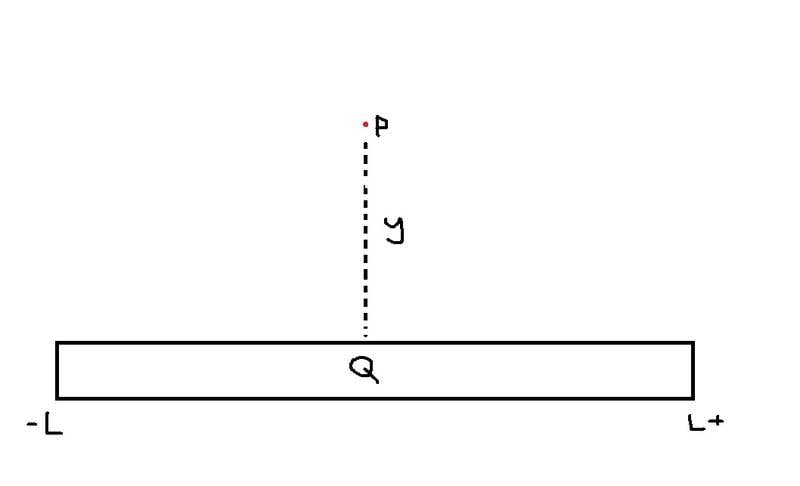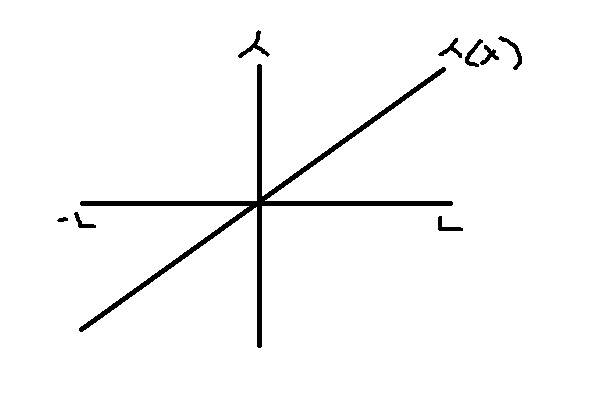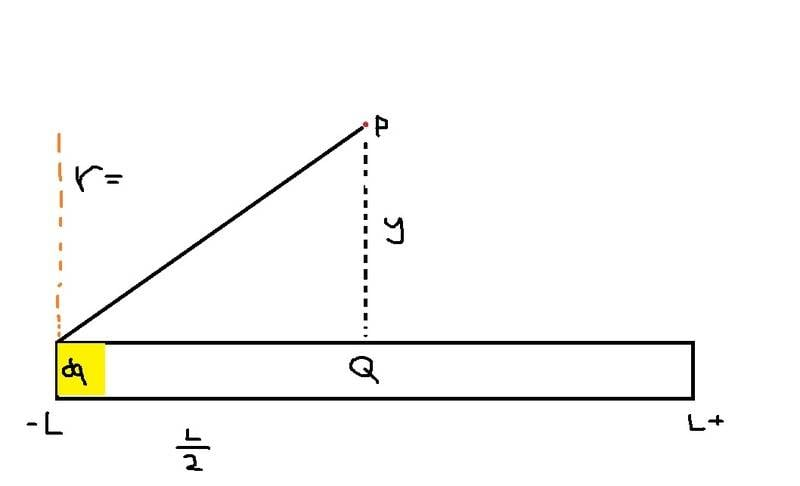# Electric potential of a uniformly charged thin rod.

freshcoast
1. Problem statementA thin rod of length 2L has charge Q uniformly distributed along its length.

1. Draw a graph of the charge density as a function of x from -L to L
2. Find the electric potential at point P a distance y away
3. Use the result in part 2 of the electric field at point P (you are finding the electric field as a function of a y equation)
4. Assess ; Draw the V versus y graph and draw the E versus y graph.

2. Known equations

v = k ∫ dq / r
e = -∇v
λ = Q / L

3. Attempt

1.graph charge density as a function of x from -L to L would just look like this because using the values and plugging it into the lambda equation it would produce these values.

2.

To find the electric potential I first need to find the correct form of dq.

Using the lambda equation
λ = Q / 2L ,

since L = 2L ,

Q = λ2L , then for dq = λ2dl

then

V = k ∫ λ2dl / √(L/2)^2 + y^2 (bounds for integral is from 0 to 2L)

because r = √(L/2)^2 + y^2

then it integrates to

$$V = 2kλ [ln(y^2 + √(L/2)^2 + y^2] |2L to 0$$

$$V = 2kλ [ln(y^2 + √((2L/2)^2 + y^2 - ln(y^2 + √(0/2)^2 + y^2))]$$

$$V = 2kλ [ln(y^2 + √L^2 + y^2 - ln(y^2 + √y^2]$$

then using log rules,

$$V = 2kλ [ln (y^2 + √L^2 + y^2 ) / (y^2 + y)$$

is this so far correct? I need to make sure this is correct before I can continue

Homework Helper
1. Problem statement

A thin rod of length 2L has charge Q uniformly distributed along its length.

1. Draw a graph of the charge density as a function of x from -L to L
2. Find the electric potential at point P a distance y away
3. Use the result in part 2 of the electric field at point P (you are finding the electric field as a function of a y equation)
4. Assess ; Draw the V versus y graph and draw the E versus y graph.
[...]
1.
[see above]
graph charge density as a function of x from -L to L would just look like this because using the values and plugging it into the lambda equation it would produce these values.
Curious - your graph has varying negative charge to the left and varying positive charge to the right .... didn't the question say that the charge "uniformly distributed"?

freshcoast
Oh, Would it just be a straight line then?

Homework Helper
You already drew a straight line though. What's important is the slope and y intercept.

If the total charge on the rod is Q, then the linear charge density of ##\lambda=Q/2L## everywhere along the rod right?

freshcoast
I was thinking the slope would be zero? so the line would be horizontal from -L to L?

Homework Helper
Now for the next step.

freshcoast
What do you think about my solution above?

Homework Helper
λ = Q / 2L , since L = 2L ,
... L=2L means that 1=2 ?!
You mean that the length of the rod is 2L?
But the charge distribution works.

V = k ∫ λ2dl / √(L/2)^2 + y^2 (bounds for integral is from 0 to 2L)
... lets see, that is:

$$V=k\int_0^{2L}\frac{\lambda 2 dl}{\sqrt{(L/2)^2+y^2}}$$
... you need to look again at this step.

You are integrating over "l": where is the "l" axis on your diagram?

You are integrating from 0 to 2L: does the rod go from 0 to 2L on your diagram?

In the integrand:
The denominator is a constant - doesn't the potential vary with distance?
The numerator has a factor of 2 in it - check the derivation of dq.

Better yet - go through the setup step by step and show me your reasoning at each stage.
Starting with: how much charge dq is on the rod between x and x+dx

Last edited:
freshcoastI set my zero point to the very left, I am confused about the length part, I know the only thing that varies would be the length, so I am thinking if I set my lambda equation as

total length of rod is 2L,

$$λ = Q / 2L$$

I isolate Q to find dq,

$$λ2L = Q$$
$$λ2dl = dq$$

I guess the 2 goes away when I derive it, so my dq equation is

$$λdl = dq$$

Which I think would work if I integrate it from 0 to 2L and having λ = Q / 2L , I would get Q back.

Now for my R, I was thinking my new equation would be

$$r = √(2L-x)^2 + y^2)$$

I am confused at the part where I know the 2L is constant, the x is the one that varies, but in my dq I have it as λdl, does it matter if the dl should be a dx or not?

But I am thinking my 2L-x is correct when I integrate it from 0 to 2L, at 2L electric potential would be 0 and when it is at 0 the electric potential would be at it's highest.

Homework Helper
If you put the x axis along the length of the rod with +x pointing to the right, and put x=0 in the center of the rod, then you get to exploit symmetry much more easily.

Anyway - your way puts x=0 on the far left, so point P is at position (L,y).
You drew your dq in an inconvenient place for visualizing what happens... put it at an arbitrary position (x,0)

So dq is the amount of charge between x and x+dx on the rod.
That's a length of (x+dx)-x=dx.

The charge per unit length is λ=Q/2L, therefore the charge in some arbitrary length Δx is Δq=λΔx... so the amount of charge dq in length dx is...

On your diagram, the distance r is the distance from dq to point P, that is the distance between point (x,0) and point (L,y) ... you have a formula for working out the distance between two points right?

freshcoast
I see... Well disregard my original attempt I will now use your suggestion to try this problem. Setting x=0 at the center where +x is to the right, I believe my dq will be

dq = (lamda)dx

Now that I have that I can complete the integral

V = k § (lamda)dx / y

Since r = y

Now I remove the constants lamda and 1/y out which leaves dx in the integral and the bounds are from -L to L

Which solves to

V = 2Lk(lamda) / y

Homework Helper
V = k § (lamda)dx / y

Since r = y
That way you'll end up with V=kQ/y ... which is the same as for a point charge.
Is that correct? The charge distribution does not matter?!

Remember what dq actually is?
It is the amount of charge between x and x+dx on the rod.
Doesn't r depend on the x position of dq as well?

Note: the integral sign is "∫" in the quick-symbols chart and \int in LaTeX.
lambda is "λ" in quick-symbols and \lambda in LaTeX

LaTeX is very useful - eg. I can write your integral as:
Code:
V=k\int_{-L}^L \frac{\lambda dx}{r(x)}
... so it comes out like: $$V=k\int_{-L}^L \frac{\lambda dx}{r(x)}$$

Last edited:
freshcoast
Hmm I see, since the charge is also a dependant of the x axis, and if I set x to be the amount of distance I'm subtracting off the whole rod...

my r would be

sqrt(L-x)^2 + y^2

Homework Helper
Hmm I see, since the charge is also a dependant of the x axis, and if

my r would be

sqrt(L-x)^2 + y^2
... you said that ##r=\sqrt{(L-x)^2}+y^2## typo? I'm guessing you meant that:

##r=\sqrt{(L-x)^2+y^2}##

... That would be if you put the origin of the coordinate system at one end of the rod.
Please make up your mind - it affects the limits of integration.

When you are setting up an integral, you need to be consistent and careful with your notation.

What you are doing is finding the contribution dV to the overall potential at P due to the charge element dq between x and x+dx on the rod. You end up with an equation dV=kλdx/r(x) - adding up all the dV's means integrating both sides... gets you V=kλ∫(dx/r(x))

freshcoast
Ok I think I can see it now,

R would be just sqrt(x^2 + y^2), now that makes sense since my bounds are from L to -L